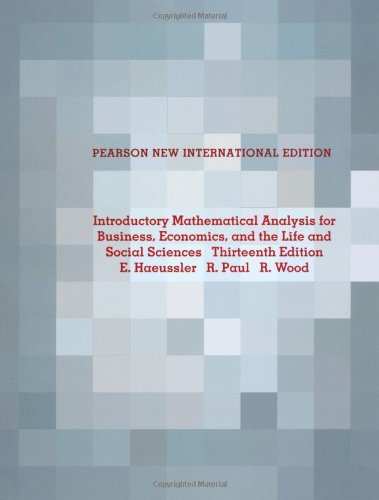### Introductory Mathematical Analysis for Business, Economics, and the Life and Social Sciences

 Yazar : Ernest F Haeussler Richard S. Paul Richard J. Wood Yayınevi : Pearson Education ISBN : 9781292021140 Baskı yılı : 2013 Sayfa sayısı : 864 Ağırlık : 1,58 kg Edisyon : 13 Stok durumu : Tükendi

This book is ideal for one- or two-semester or two- or three-quarter courses covering topics in college algebra, finite mathematics, and calculus for students in business, economics, and the life and social sciences. Haeussler, Paul, and Wood establish a strong algebraic foundation that sets this text apart from other applied mathematics texts, paving the way for students to solve real-world problems that use calculus. Emphasis on developing algebraic skills is extended to the exercises-including both drill problems and applications. The authors work through examples and explanations with a blend of rigor and accessibility. In addition, they have refined the flow, transitions, organization, and portioning of the content over many editions to optimize manageability for teachers and learning for students. The table of contents covers a wide range of topics efficiently, enabling instructors to tailor their courses to meet student needs.
Part I. ALGEBRA 0. Review of Algebra 0.1 Sets of Real Numbers 0.2 Some Properties of Real Numbers 0.3 Exponents and Radicals 0.4 Operations with Algebraic Expressions 0.5 Factoring 0.6 Fractions 0.7 Equations, in Particular Linear, Equations 0.8 Quadratic Equations 1. Applications and More Algebra 1.1 Applications of Equations 1.2 Linear Inequalities 1.3 Applications of Inequalities 1.4 Absolute Value 1.5 Summation Notation 1.6 Sequences 2. Functions and Graphs 2.1 Functions 2.2 Special Functions 2.3 Combinations of Functions 2.4 Inverse Functions 2.5 Graphs in Rectangular Coordinates 2.6 Symmetry 2.7 Translations and Reflections 2.8 Functions of Several Variables 3. Lines, Parabolas, and Systems 3.1 Lines 3.2 Applications and Linear Functions 3.3 Quadratic Functions 3.4 Systems of Linear Equations 3.5 Nonlinear Systems 3.6 Applications of Systems of Equations 4. Exponential and Logarithmic Functions 4.1 Exponential Functions 4.2 Logarithmic Functions 4.3 Properties of Logarithms 4.4 Logarithmic and Exponential Equations Part II. FINITE MATHEMATICS 5. Mathematics of Finance 5.1 Compound Interest 5.2 Present Value 5.3 Interest Compounded Continuously 5.4 Annuities 5.5 Amortization of Loans 5.6 Perpetuities 6. Matrix Algebra 6.1 Matrices 6.2 Matrix Addition and Scalar Multiplication 6.3 Matrix Multiplication 6.4 Solving Systems by Reducing Matrices 6.5 Solving Systems by Reducing Matrices (continued) 6.6 Inverses 6.7 Leontiefs Input-Output Analysis 7. Linear Programming 7.1 Linear Inequalities in Two Variables 7.2 Linear Programming 7.3 Multiple Optimum Solutions 7.4 The Simplex Method 7.5 Degeneracy, Unbounded Solutions, and Multiple Solutions 7.6 Artificial Variables 7.7 Minimization 7.8 The Dual 8. Introduction to Probability and Statistics 8.1 Basic Counting Principle and Permutations 8.2 Combinations and Other Counting Principles 8.3 Sample Spaces and Events 8.4 Probability 8.5 Conditional Probability and Stochastic Processes 8.6 Independent Events 8.7 Bayess Formula 9. Additional Topics in Probability 9.1 Discrete Random Variables and Expected Value 9.2 The Binomial Distribution 9.3 Markov Chains Part III. CALCULUS 10. Limits and Continuity 10.1 Limits 10.2 Limits (Continued) 10.3 Continuity 10.4 Continuity Applied to Inequalities 11. Differentiation 11.1 The Derivative 11.2 Rules for Differentiation 11.3 The Derivative as a Rate of Change 11.4 The Product Rule and the Quotient Rule 11.5 The Chain Rule 12. Additional Differentiation Topics 12.1 Derivatives of Logarithmic Functions 12.2 Derivatives of Exponential Functions 12.3 Elasticity of Demand 12.4 Implicit Differentiation 12.5 Logarithmic Differentiation 12.6 Newtons Method 12.7 Higher-Order Derivatives 13. Curve Sketching 13.1 Relative Extrema 13.2 Absolute Extrema on a Closed Interval 13.3 Concavity 13.4 The Second-Derivative Test 13.5 Asymptotes 13.6 Applied Maxima and Minima 14. Integration 14.1 Differentials 14.2 The Indefinite Integral 14.3 Integration with Initial Conditions 14.4 More Integration Formulas 14.5 Techniques of Integration 14.6 The Definite Integral 14.7 The Fundamental Theorem of Integral Calculus 14.8 Approximate Integration 14.9 Area between Curves 14.10 Consumers and Producers Surplus 15. Methods and Applications of Integration 15.1 Integration by Parts 15.2 Integration by Partial Fractions 15.3 Integration by Tables 15.4 Average Value of a Function 15.5 Differential Equations 15.6 More Applications of Differential Equations 15.7 Improper Integrals 16. Continuous Random Variables 16.1 Continuous Random Variables 16.2 The Normal Distribution 16.3 The Normal Approximation to the Binomial Distribution 17. Multivariable Calculus 17.1 Partial Derivatives 17.2 Applications of Partial Derivatives 17.3 Implicit Partial Differentiation 17.4 Higher-Order Partial Derivatives 17.5 Chain Rule 17.6 Maxima and Minima for Functions of Two Variables 17.7 Lagrange Multipliers 17.8 Lines of Regression 17.9 Multiple Integrals